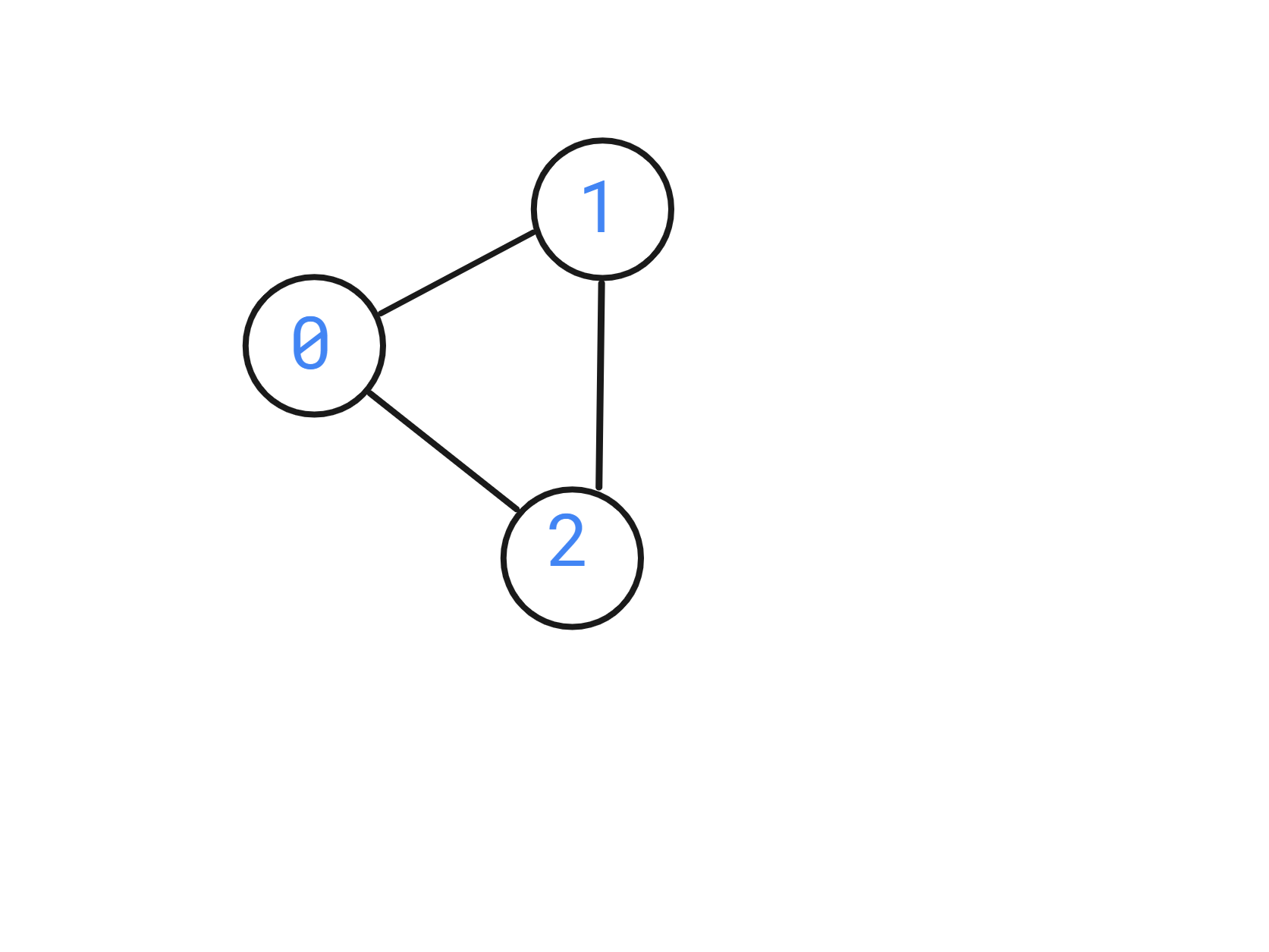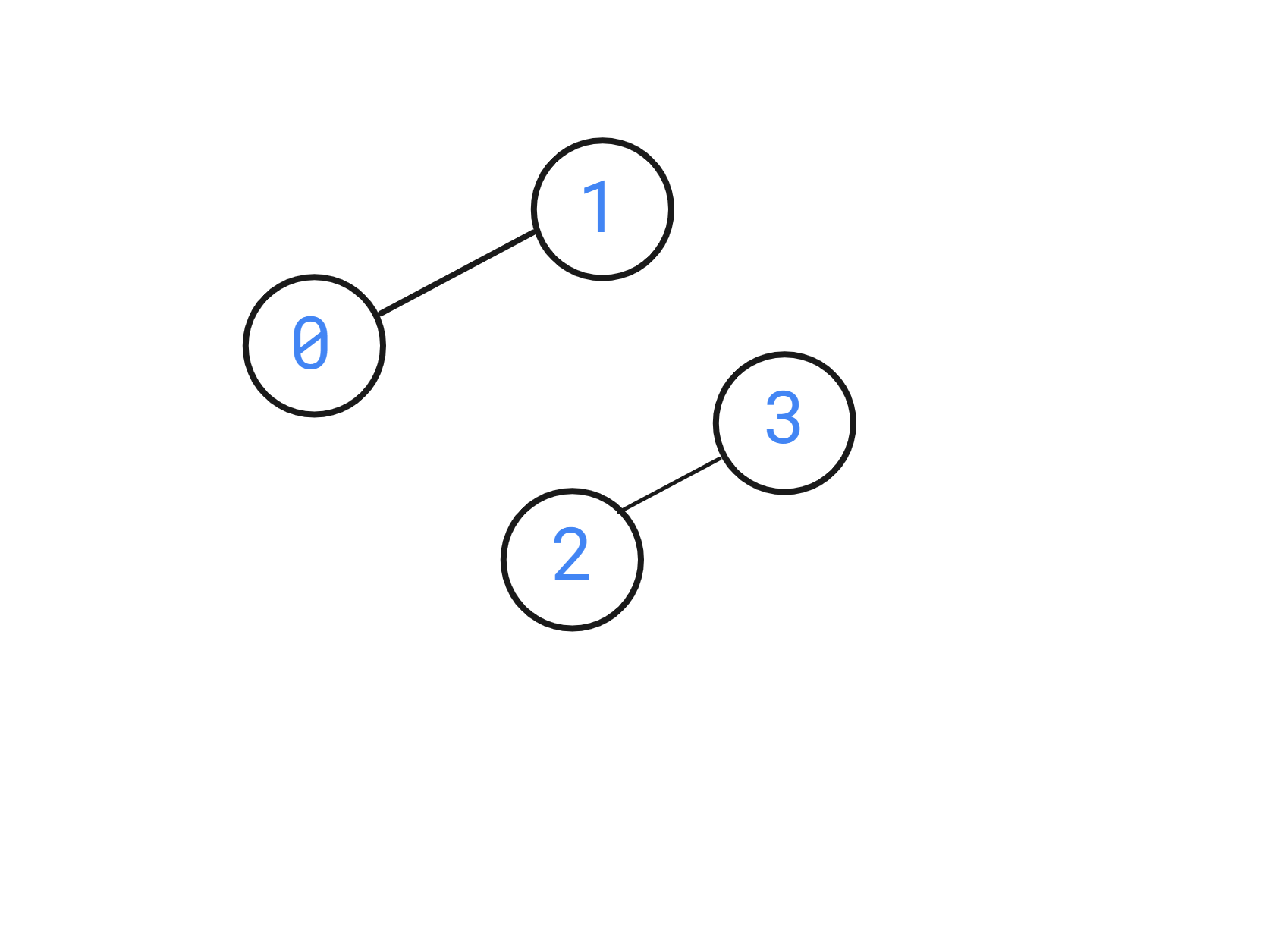24
Avg. time to solve
50 min
Success Rate
50%

Problem Statement

You are given a 2D array ‘edges’ which contains 0 and 1, where ‘edges[i][j]’ = 1 denotes a bi-directional edge from ‘i’ to ‘j’.

Note:
If edges[i][j] = 1, that implies there is a bi-directional edge between ‘i’ and ‘j’, that means there exists both edges from ‘i’ to ‘j’ and to ‘j’ to ‘i’.

For example

Given:
‘N’ = 3
‘edges’ = [[0, 1, 1], [0, 0, 1], [0,0,0]].Input format:
The first line of input contains an integer ‘T’ denoting the number of test cases.

The first line of each test case contains two space-separated integers, ‘N,’ where ‘N’ is the number of rows in ‘edges’ and the number of columns in ‘edges’.

The next ‘N’ line of each test case contains ‘N’ space-separated integers which tell if there is an edge between ‘i’ and ‘j’.
Output Format :
For each test case, You are supposed to return a bool value determining whether the graph is bipartite or not.
Note:
You are not required to print the expected output; it has already been taken care of. Just implement the function.
Constraints:
1 <= ‘T’ <= 10
2 <= ‘N’ <= 300
0 <= ‘edges[i][j]’ <= 1.

Time Limit: 1sec.

2
4
0 1 0 0
0 0 0 1
0 0 0 0
0 0 0 0
3
0 1 1
0 0 1
0 0 0

1
0

Explanation of the Sample Input 1:

In the first test case, the graph is visualized as below,The graph can be divided into 2 disjointed sections, i.e. S1 = {0,2} and S2 = {1,3}. Therefore the answer is true.

In the second test case, the graph is visualized as below:The answer is 0 since there is no way this graph can be divided into 2 disjoint sets of points.

2
4
0 0 1 0
0 0 0 1
0 0 0 0
0 0 0 0
3
0 1 1
0 0 0
0 0 0

Sample Output 2 :

1
1Console